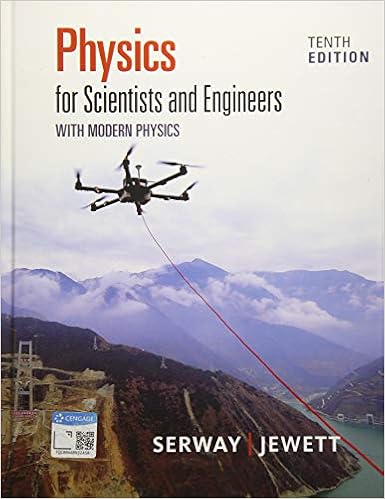# Pts at a circus a clown on a motorcycle with a mass m

• Test Prep
• 9

This preview shows page 4 - 6 out of 9 pages.

##### We have textbook solutions for you!
The document you are viewing contains questions related to this textbook.The document you are viewing contains questions related to this textbook.
Chapter 2 / Exercise 2.1
Physics for Scientists and Engineers with Modern Physics
Jewett/SerwayExpert Verified
9. (6 pts.) At a circus, a clown on a motorcycle with a mass M travels along a horizontal track and enters a vertical circle of radius r . Which one of the following expressions determines the minimum speed that the motorcycle must have at the top of the track to remain in contact with the track? A. B. C. v = gR D. v = 2 gR E. v = MgR shared via CourseHero.com 10. (6 pts.) Note the following three cases. In which case will the magnitude of the normal force on the block be equal to ( Mg + F sin θ )? 11. (6 pts.) The figure gives the angular momentum L of a wheel versus time t. Which of the following is true for the magnitudes of the torques in the intervals shown? A A A A D 12. (6 pts.): A bullet is aimed at a target on the wall a distance L away from the firing position. Because of gravity, the bullet strikes the wall a distance Δ y below the mark as suggested in the figure. Note: The drawing is not to scale. If the distance L were half as large, and the bullet had the same initial velocity, how would Δ y be affected? 2 v gr = v gr = This study resource was shared via CourseHero.com
##### We have textbook solutions for you!
The document you are viewing contains questions related to this textbook.The document you are viewing contains questions related to this textbook.
Chapter 2 / Exercise 2.1
Physics for Scientists and Engineers with Modern Physics
Jewett/SerwayExpert Verified
Problem 1 (25 pts.): A bowling ball of mass M and radius R ( treated as a uniform solid sphere is thrown along a level floor with kinetic energy K 0 . It is thrown so that initially (at t = 0) it slides with a linear speed ν 0 but does not rotate. As the ball slides, kinetic friction causes it to begin spinning. Eventually the ball begins to roll without slipping along the floor, with linear speed ν and angular speed ω , as shown below. The coefficient of kinetic friction between the ball and the floor is μ k a) ( 15 pts. ) At what time t > 0 does the bowling ball begin rolling smoothly along the floor? What is the linear speed ν at that time? Express your answers in terms of ν 0 , g, and μ k needed. b) ( 10 pts. ) What is the change Δ K in the bowling ball’s kinetic energy during this entire process ( i.e ., from sliding motion to rolling without slipping)? Express your answer in terms of K 0 This study resource was shared via CourseHero.com ) . , as .
•••